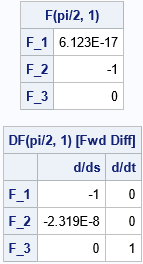I previously showed how to use SAS to compute finite-difference derivatives for smooth scalar-valued functions of several variables. You can use the NLPFDD subroutine in SAS/IML software to approximate the gradient vector (first derivatives) and the Hessian matrix (second derivatives). The computation uses finite-difference derivatives to approximate the derivatives.

The NLPFDD subroutine also supports approximating the first derivatives a smooth vector-valued function. If F: Rn → Rm is a vector-valued function with m components, then you can write it as F(x) = (F1(x), F2(x), ..., Fm(x)). The Jacobian matrix, J, is the matrix whose (i,j)th entry is J[i,j] = ∂Fi / ∂xj. That is, the i_th row is the gradient of the i_th component function, Fi, i=1,2,..,m and j=1,2...,n. The NLPFDD subroutine enables you to approximate the Jacobian matrix at any point in the domain of F.

### An example of a vector-valued function

A simple example of a vector-valued function is the parameterization of a cylinder, which is a mapping from R2 → R3 given by the function
C(s,t) = (cos(s), sin(s), t)
For any specified values of (s,t), the vector C(s,t) is the vector from the origin to a point on the cylinder. It is sometimes useful to consider basing the vectors at a point q, in which case the function F(s,t) = C(s,t) - q is the vector from q to a point on the cylinder. You can define this function in SAS/IML by using the following statements. You can then use the NLPFDD subroutine to compute the Jacobian matrix of the function at any value of (s,t), as follows:

```/* Example of a vector-valued function f: R^n -> R^m for n=2 and m=3. Map from (s,t) in [0, 2*pi) x R to the cylinder in R^3. The vector is from q = (0, 2, 1) to C(s,t) */ proc iml;   q = {0, 2, 1}; /* define a point in R^3 */ /* vector from q to a point on a cylinder */ start VecF(st) global(q); s = st; t = st; F = j(3, 1, .); F = cos(s) - q; F = sin(s) - q; F = t - q; return( F ); finish;   /* || VecF || has local min at (s,t) = (pi/2, 1), so let's evaluate derivatives at (pi/2, 1) */ pi = constant('pi'); x = pi/2 || 1; /* value of (s,t) at which to evaluate derivatives */ /* Parameters: the function has 3 components, use forward difference, choose step according to scale of F */ parm = {3, 00, .}; call nlpfdd(f, J, JtJ, "VecF", x) par=parm; /* return f(x), J=DF(x), and J`*J */   print f[r={'F_1' 'F_2' 'F_3'} L='F(pi/2, 1)'], J[r={'F_1' 'F_2' 'F_3'} c={'d/ds' 'd/dt'} L='DF(pi/2, 1) [Fwd Diff]'];```The program evaluates the function and the Jacobian at the point (s,t)=(π/2, 1). You must use the PAR= keyword when the function is vector-valued. The PAR= argument is a three-element vector that contains the following information. If you specify a missing value, the default value is used.

• The first element is the number of components (m) for the function. By default, the NLPFDD subroutine expects a scalar-valued function (m=1), so you must specify PARM=3 for this problem.
• The second element is the finite-difference method. The function supports several schemes, but I always use either 0 (forward difference) or 1 (central difference). By default, PARM=0.
• The third element provides a way to choose the step size for the finite-difference method. I recommend that you use the default value.

The output shows that the value of the function at (s,t)=(π/2, 1) is {0, -1, 0}. The Jacobian at that point is shown. The gradient of the i_th component function (Fi) is contained in the i_th row of the Jacobian matrix.

• The gradient of the first component function at (π/2, 1) is {-1 0}.
• The gradient of the second component function at (π/2, 1) is {0 0}, which means that F2 is at a local extremum. (In this case, F2 is at a minimum).
• The gradient of the third component function is {0 1}.

The NLPFDD subroutine also returns a third result, which is named JtJ in the program. It contains the matrix product J`*J, where J is the Jacobian matrix. This product is used in some numerical methods, such as the Gauss-Newton algorithm, to minimize the value of a vector-valued function. Unless you are writing your own algorithm to perform a least-squares minimization, you probably won't need the third output argument.

### Summary

The NLPFDD subroutine in SAS/IML uses finite-difference derivatives to approximate the derivatives of multivariate functions. A previous article shows how to use the NLPFDD subroutine to approximate the gradient and Hessian of a scalar-valued function. This article shows how to approximate the Jacobian of a vector-valued function. Numerical approximations for derivatives are useful in all sorts of numerical routines, including optimization, root-finding, and solving nonlinear least-squares problems.

In SAS Viya, you can use the FDDSOLVE subroutine, which is a newer implementation that has a similar syntax.

Share